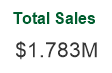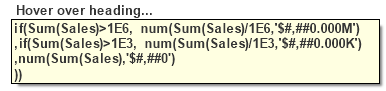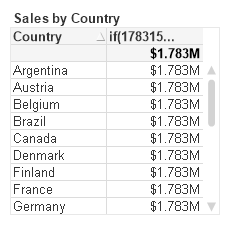# Comment formater le type monnaie ?

0
584

SummaryI demonstrate a simple reusable expression to auto scale numbers in QlikView. This leads to an exploration of some of the finer details of dollar sign expansion.

The QlikView auto-scaling feature selects an appropriate unit – billion, million, thousands — based on the magnitude of the  Y-axis values.  It’s a nice feature  available in Line and Bar charts.  How can we create the same functionality in Text Objects or Straight Tables?

It’s easy enough to use an if() function that tests the magnitude, does any necessary division, and formats appropriately. For example:

```if(Sum(Sales)>1E6, num(Sum(Sales)/1E6,'\$#,##0.000M')
,if(Sum(Sales)>1E3, num(Sum(Sales)/1E3,'\$#,##0.000K')
,num(Sum(Sales),'\$#,##0')
))```

(The 1E6 is an easier way to write 1000000).

To avoid repeated coding of “Sum(Sales)” I create a reusable variable with parameters in the script like this:

```SET vScaleNumber=if(\$1>1E6, num(\$1/1E6,'\$#,##0.000M')
,if(\$1>1E3, num(\$1/1E3,'\$#,##0.000K')
,num(\$1,'\$#,##0')
));```

Now I can use the variable vScaleNumber in a Text Object as:

`=\$(vScaleNumber(Sum(Sales)))`

The string “Sum(Sales)” will get substituted in  every occurrence of “\$1”.  I ‘ll get an appropriately formatted number like:If I use “\$(vScaleNumber(Sum(Sales)))” in a Straight Table expression without label, hovering over the column heading will show me the full substitution in  a tooltip.I  can see that the “\$1” substitution occurs before the expression is evaluated, and the substituted expression looks like:

```if(Sum(Sales)>1E6, num(Sum(Sales)/1E6,'\$#,##0.000M')
,if(Sum(Sales)>1E3, num(Sum(Sales)/1E3,'\$#,##0.000K')
,num(Sum(Sales),'\$#,##0')
))```

I’ve avoided re-typing “Sum(Sales)”. But I may have a concern about the performance implications of repeated execution of “Sum(Sales)”.  And what about more complex expressions such as “Round(Sum(Sales),10)”?  The comma in that expression will break the syntax as variable parameters always treat commas as parameter separators.I can fix the comma/performance problem by using Dollar Sign Expansion (DSE) with an “=”.  The “=” will cause the expression to evaluate and pass the numerical result to vScaleNumber.

`=\$(vScaleNumber(\$(=round(Sum(Sales),10))))`

Checking the expansion in a Straight Table shows:

```if(1783150>1E6, num(1783150/1E6,'\$#,##0.000M')
,if(1783150>1E3, num(1783150/1E3,'\$#,##0.000K')
,num(1783150,'\$#,##0')
))```

I  see the value of “round(Sum(Sales),10)” has been calculated as “1783150”,  yielding an efficient and syntactically correct expression.

Next  I’ll add a Dimension to the Straight Table.  The row results are incorrect!The “=” in the DSE caused the Sum expression  to be evaluated only once for the entire chart, yielding the same value for every row.  How to fix?

I will calculate the sum() expression in a n Expression n column, and then hide this column on the Presentation tab. I can then refer to the hidden column:

`=\$(vScaleNumber(Column(1)))`

Once again, the expansion yields an efficient and syntactically correct expression.

```if(Column(1)>1E6, num(Column(1)/1E6,'\$#,##0.000M')
,if(Column(1)>1E3, num(Column(1)/1E3,'\$#,##0.000K')
,num(Column(1),'\$#,##0')
))```

I started this post by demonstrating a simple expression to format scaled numbers. It’s a function I frequently use.

For more on DSE, see Henric’s post at https://community.qlik.com/blogs/qlikviewdesignblog/2013/11/18/dollar-expansions